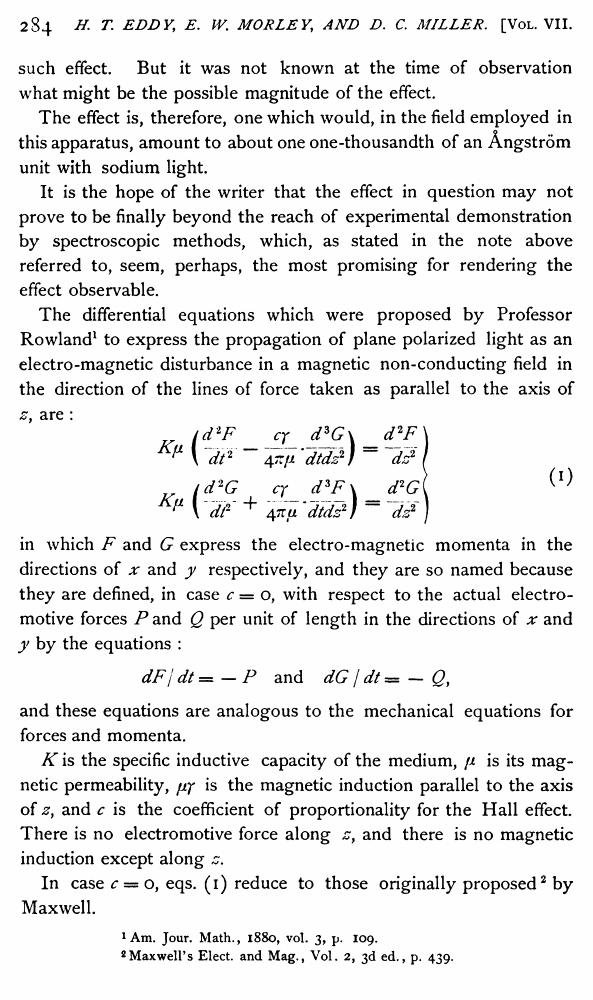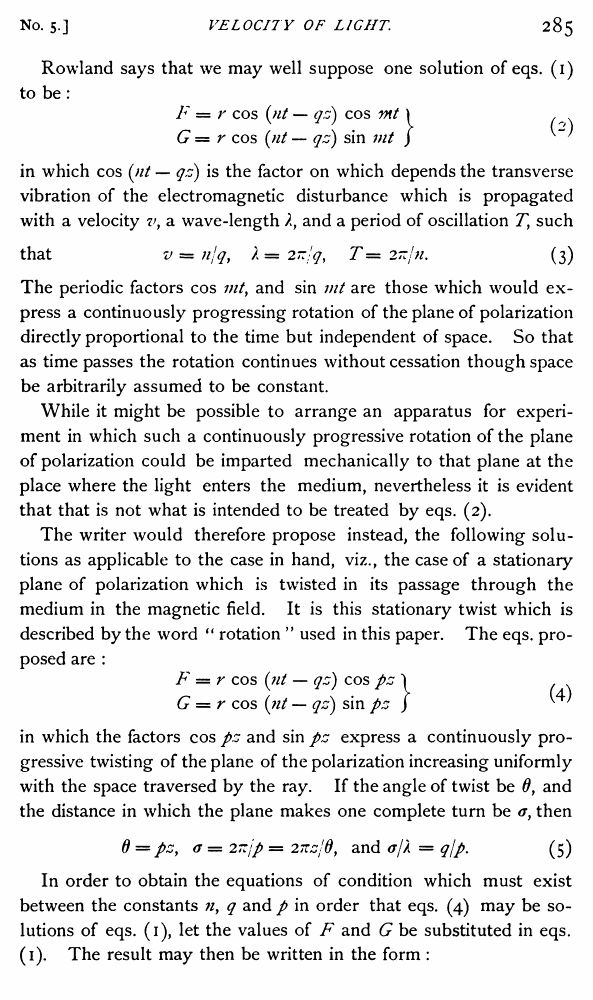Henry T. Eddy, Edward W. Morley, Dayton C. Miller. The Velocity of Light in The Magnetic Field //Physical Review (Series I), Volume 7, Issue 5 (1898)

В начало   Другие форматы   <<<     Страница 284   >>>283  284 285  286  287  288  289  290  291  292  293  294  295  296 such effect. But it was not known at the time of observation what might be the possible magnitude of the effect. The effect is, therefore, one which would, in the field employed in this apparatus, amount to about one one-thousandth of an Angstrom unit with sodium light. It is the hope of the writer that the effect in question may not prove to be finally beyond the reach of experimental demonstration by spectroscopic methods, which, as stated in the note above referred to, seem, perhaps, the most promising for rendering the effect observable. The differential equations which were proposed by Professor Rowland1 to express the propagation of plane polarized light as an electro-magnetic disturbance in a magnetic non-conducting field in the direction of the lines of force taken as parallel to the axis of z, are : id'lF cy_ d*G\ _d2F\ P \ dt2 ~ ~4t:Ji'dtdz2) ~ ~dz2 I id2G cr_ dsF\ _ d2G\ ^ " \ dt-' + 4TtU 'dtds2) ~ ~d?) in which F and G express the electro-magnetic momenta in the directions of x and y respectively, and they are so named because they are defined, in case c = o, with respect to the actual electromotive forces P and Q per unit of length in the directions of x and y by the equations : dF j dt = — P and dG / dt = — Qf and these equations are analogous to the mechanical equations for forces and momenta. K is the specific inductive capacity of the medium, fi is its magnetic permeability, fiy is the magnetic induction parallel to the axis of zy and c is the coefficient of proportionality for the Hall effect There is no electromotive force along zy and there is no magnetic induction except along z. In case c = o, eqs. (1) reduce to those originally proposed2 by Maxwell. 1 Am. Jour. Math., 1880, vol. 3, p. 109. 2Maxwell’s Elect, and Mag., Vol. 2, 3d ed., p. 439. Rowland says that we may well suppose one solution of eqs. (1) to be : F = r cos (nt — qz) cos vit \ , G = r cos (nt — qz) sin mt ) in which cos (nt — qz) is the factor on which depends the transverse vibration of the electromagnetic disturbance which is propagated with a velocity vy a wave-length and a period of oscillation Tf such that v = njq, / = T — 2~jn. (3) The periodic factors cos mt, and sin mt are those which would express a continuously progressing rotation of the plane of polarization directly proportional to the time but independent of space. So that as time passes the rotation continues without cessation though space be arbitrarily assumed to be constant. While it might be possible to arrange an apparatus for experiment in which such a continuously progressive rotation of the plane of polarization could be imparted mechanically to that plane at the place where the light enters the medium, nevertheless it is evident that that is not what is intended to be treated by eqs. (2). The writer would therefore propose instead, the following solutions as applicable to the case in hand, viz., the case of a stationary plane of polarization which is twisted in its passage through the medium in the magnetic field. It is this stationary twist which is described by the word “ rotation ” used in this paper. The eqs. proposed are : F — r cos (tit — qz) cos pz 'I G = r cos (;// — qz) sin pz ) ^ ' in which the factors cos pz and sin pz express a continuously progressive twisting of the plane of the polarization increasing uniformly with the space traversed by the ray. If the angle of twist be 0f and the distance in which the plane makes one complete turn be